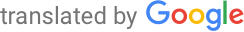Machine-translated page for increased accessibility for English questioners.

# Theoretical informatics

1. Probability. Basic concepts and properties of probability; random variable and its characteristics (mean, variance, moments, ....) and their use; binomial, geometric and other distributions and their characteristics. Markov and Chebyshev inequality and their use; Chernoff's estimates and their use; random, especially Markov, processes and their properties; entropy and information and their properties; applications in informatics.
`IV111, IA062`
2. Algebra. Linear algebra; vector and matrix calculus; groups, circuits and arrays and their basic properties; unions and their basic properties; distributive, modular and Boolean unions; universal algebras (basic concepts, homomorphisms, congruences and factor algebras), varieties, Birkhoff's theorem.
`MA009`
3. Graphs and graph algorithms. Basic concepts and methods of representation and search; trees and their representation and search; planar graphs and their properties, algorithms for minimal skeleton construction, for finding the shortest path; for optimal pairing; for maximum flows, etc .; data structures for graph algorithms; random graphs and their use; random graph algorithms.
`MA015, MA010`

## Focus Algorithms

1. Vending machines. Finite deterministic, nondeterministic and probabilistic automata and their properties (minimization, equivalence, decision problems); regular languages and their properties; pumping lemma, stack automata and their properties; context-free languages and their properties; Chomsky grammars and their normal forms and relation to automata models. Syntactic analysis of the most used models of context-free grammars; finite automata over infinite words; Turing machines and their properties (recursive and recursively countable sets, universality and halting problem).
2. Computational, communication and Kolmogorov complexity. Time and memory complexities and their properties; Complexity classes for deterministic, nondeterministic and random calculations and the relationships between them; complete, especially NP-complete, problems; evidence of NP-completeness; P-NP problem and its implications; approximate solution of NP-problems; approximation complexity classes; interactive evidence systems and zero-knowledge evidence systems; communication complexity and its applications; methods of proving lower estimates of communication complexity; classes of communication complexity, basic concepts and properties of Kolmogorov complexity.
`IA012, IA062`
3. Algorithms for solving computationally demanding problems. Monte Carlo and Las Vegas random algorithms; random walks; approximation algorithms and their potential; genetic algorithms; quantum algorithms (Shor's factorization and Grover's search algorithm).
`IA101, IA062`
4. Coding, cryptography and cryptographic protocols. Linear codes (definition, properties, coding and decoding, important examples of linear codes; cyclic codes (definition, algebraic characterization, coding and decoding, important examples of cyclic codes); turbo codes and low-density codes; private key cryptosystems; DES and AES cryptosystems, public key cryptosystems, RSA cryptosystem and its properties, cryptographic systems based on elliptic curves, schemes for digital signatures, authentication protocols, protocols for coin tossing, bit commitment and oblivious transfer, steganography and watermarking, cryptographic machines and their history, quantum cryptography (generation of random secret classical keys, quantum teleportation).
`IV054`
5. Mathematical analysis. Systems of linear differential equations and methods of their solution; curve integral.
`MA002`

## Focus Logic

1. Mathematical logic and computability. Propositional logic (propositional formulas, truth, provability, completeness theorem); predicate logic (axioms, semantics, provability); theorems on correctness, completeness, deduction and compactness; theories and models; Löwenheim-Skolem theorem; Gödel's theorems on completeness and incompleteness.
2. Rice's theorems; creative and immune sets; primitive, totally and partially recursive sets and functions. Arithmetic sets and functions; Gödel's and Rosser's incompleteness theorem; Gödel's second incompleteness theorem.
`MA007, IA038, IA046`
3. Semantics of programming languages. Basic approaches to the semantics of programming languages (operational, denotative and axiomatic); structural operational semantics and its variants; semantic equivalence; basic concepts and methods of denotative semantics (concept of CPO, continuous functions between CPOs, fixed point theorem, semantics of recursion); equivalence of denotation and operational semantics.
`IA011`
4. Petri nets. Basic properties of Petri nets (boundedness, coverability, Karp-Miller tree, weak Petri computer, reachability and lifetime); (in) decidability of semantic equivalences and temporal logics for Petri nets; S-systems and S-variants, T-systems and T-variants; Free choice petri nets; modeling using Petri nets.
`IA023`
5. Formal verification methods. Main verification methods and their comparison; state explosion problem; theorem proving as a deductive verification method; LTL-model checking for finite and infinite state systems; bounded model checking; symbolic execution; static analysis; abstract interpretation; verification means.
`IV113, IA159`
6. Process theory, specifications and logic. Processes and transition systems with labels and their specifications; operational semantics; process hierarchy; basic semantic equivalences of processes on transition systems and their mutual relations; possibilities of algorithmic verifiability of semantic equivalences on infinite state processes. Modal logics (propositional modal logic, modal mu-calculus); temporal logics (temporal operators, propositional temporal logic, linear and branching time); processes and their properties; verification of temporal properties; model checking; automated verification.
`IA040, IA041`
7. Lambda calculus. Elements of lambda calculus; transformation and reduction; lambda-calculus and calculations (coding, recursive definitions, lambda-computability, undecidable problems); typed lambda-calculus (properties and applications); domains and their construction; domain models.
`IA081`